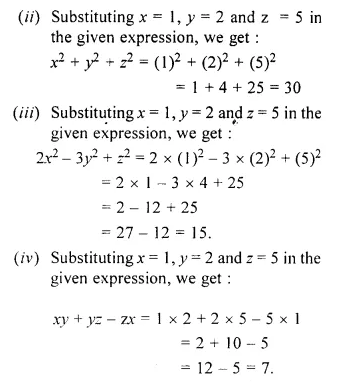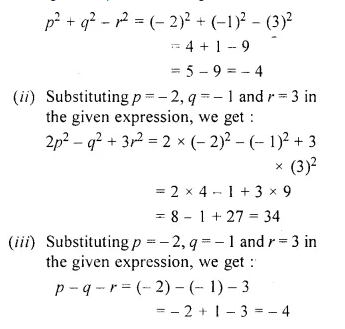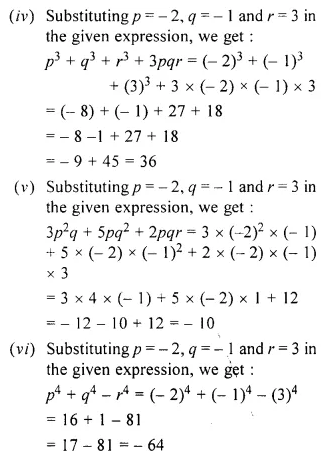## RS Aggarwal Class 6 Solutions Chapter 8 Algebraic Expressions Ex 8B

These Solutions are part of RS Aggarwal Solutions Class 6. Here we have given RS Aggarwal Solutions Class 6 Chapter 8 Algebraic Expressions Ex 8B.

Other Exercises

Question 1.
Solution:
(i) Substituting a = 2 and b = 3 in the , given expression, we get :
a + b = 2 + 3 = 5
(ii) Substituting a = 2 and b = 3 in the given expression, we get :
a2 + ab = (2)2 + 2 x 3
= 4 + 6 = 10
(iii) Substituting a = 2 and b = 3 in the given expression, we get :
ab – a2 = 2 x 3 – (2)2
= 6 – 4 = 2
(iv) Substituting a = 2 and b = 3 in the given expression, we get :
2a – 3b = 2 x 2 – 3 x 3
= 4 – 9 = – 5
(v) Substituting a = 2 and b = 3 in the given expression, we get :
5a2 – 2ab = 5 x (2)2 – 2 x 2 x 3
= 5 x 4 – 4 x 3
= 20 – 12 = 8
(vi) Substituting a = 2 and b = 3 in the given expression, we get :
a3 – b3 = (2)3 – (3)3 = 2 x 2 x 2 – 3 x 3 x 3
= 8 – 27 = – 19

Question 2.
Solution:
(i) Substituting x = 1, y = 2 and z = 5 in the given expression, we get :
3x – 2y + 4z = 3 x 1 – 2 x 2 + 4 x 5
= 3 – 4 + 20 = 23 – 4 = 19Question 3.
Solution:
(i) Substituting p = – 2, q = – 1 and r = 3
in the given expression, we get :Question 4.
Solution:
(i) The coefficient of x in 13x is 13
(ii) The coefficient of y in – 5y is – 5
(iii) The coefficient of a in 6ab is 6b
(iv) The coefficient of z in – 7xz is – 7x
(v) The coefficient of p in – 2pqr is – 2qr
(vi) The coefficient of y2 in 8xy2z is 8xz
(vii) The coefficient of x3 in x3 is 1
(viii) The coefficient of x2 in – x2 is -1

Question 5.
Solution:
(i) The numerical coefficient of ab is 1
(ii) The numerical coefficient of – 6bc is – 6
(iii) The numerical coefficient of 7xyz is 7
(iv) The numerical coefficient of – 2x3y2z is – 2.

Question 6.
Solution:
(i) The constant term is 8
(ii) The constant term is – 9
(iii) The constant term is $$\\ \frac { 3 }{ 5 }$$
(iv) The constant term is $$– \frac { 8 }{ 3 }$$

Question 7.
Solution:
(i) The given expression contains only one term, so it is monimial.
(ii) The given expression contains only two terms, so it is binomial.
(iii) The given expression contains only one term, so it is monomial.
(iv) The given expression contains three terms, so it is trinomial.
(v) The given expression contains three terms, so it is trinomial.
(vi) The given expression contains only one term, so it is monomial.
(vii) The given expression contains four terms, so it is none of monomial, binomial and trinomial.
(viii) The given expression contains only one term so it is monomial.
(ix) The given expression contains two terms, so it is binomial.

Question 8.
Solution:
(i) The terms of the given expression 4x5 – 6y4 + 7x2y – 9 are :
4x5, – 6y4, 7x2y, – 9
(ii) The terms of the given expression 9x3 – 5z4 + 7x3y – xyz are :
9x3, – 5z4, 7x3y, – xyz.

Question 9.
Solution:
(i) We have : a2, b2, – 2a2, c2, 4a
Here like terms are a2, – 2a2
(ii) We have : 3x, 4xy, – yz, $$\\ \frac { 1 }{ 2 }$$ zy
Here like terms are – yz, $$\\ \frac { 1 }{ 2 }$$ zy
(iii) We have : – 2xy2, x2y, 5y2x, x2z
Here like terms are – 2xy2, 5y2x
(iv) We have :
abc, ab2c, acb2, c2ab, b2ac, a2bc, cab2
Here like terms are ab2c, acb2, b2ac, cab2.

Hope given RS Aggarwal Solutions Class 6 Chapter 8 Algebraic Expressions Ex 8B are helpful to complete your math homework.

If you have any doubts, please comment below. Learn Insta try to provide online math tutoring for you.